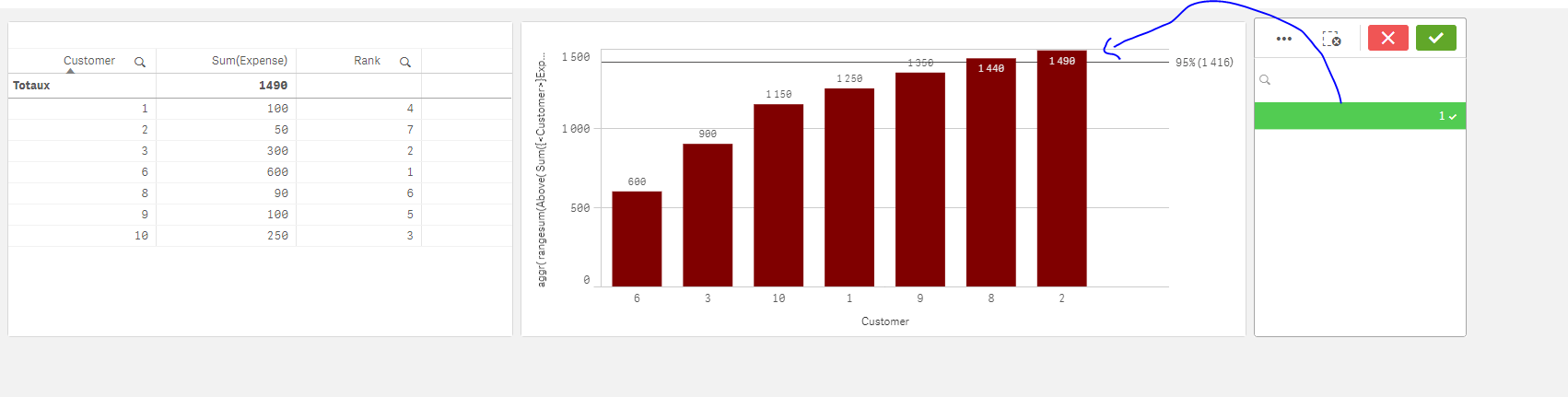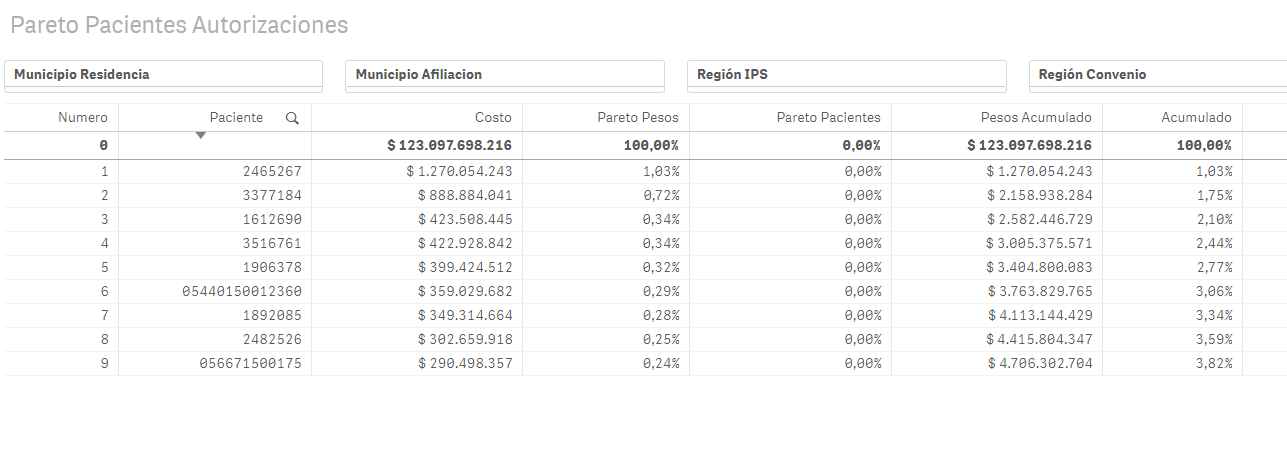# New to Qlik Sense

If you’re new to Qlik Sense, start with this Discussion Board and get up-to-speed quickly.

Announcements
Skip the ticket, Chat with Qlik Support instead for instant assistance.
cancel
Showing results for
Did you mean:Contributor III

## Select the 1% of the most expensive population

Hi,

7 RepliesMVP

Can u please share what u have?

some data?

It would be much easier to imagine a solution when we know how the data is constructed/calculatedThanksContributor III
Author

Hi Omar,

My data is structurate for servises then i have to aggregate the data for paciente, like that aggr(Sum(Costo),Paciente)MVP

Can u dos thing like :

here's what I have:

CountryMeasure

and here's what I want to do : ...

?Specialist III

Have you tried looking at this?  It seems like it would set you off in the right direction.  And don't forget to give us some example data to help you with.  Omar is really, really good and helpful if you give him something to work with.

Recipe for a Pareto AnalysisMVP

STill don't know what u want to do; but I'll be trying to imagine that u have this and u want a pareto :

1) I'll add a rank field in my script

t:

Customer, Expense

1,100

2,50

3,300

4,40

5,50

6,600

7,10

8,90

9,100

10,250

];

t0:

load Customer, sum(Expense) as sum Resident t Group by Customer ;

NoConcatenate

load Customer Resident t0 Order by sum desc;

Drop table t0;

2)
Create a bar chart

as dimension : Customer

as measure:

aggr(

rangesum(Above(

Sum({<Customer>}Expense)

, 0 , RowNo()))

,Rank)

sort it with expression : Rank (Ascend)

color it by expression:

if(aggr(

rangesum(Above(

Sum({<Customer>}Expense)

, 0 , RowNo()))

,Rank) / sum(total {<Customer>} Expense) <=0.95,red())

Result:Now as a filter, create it as follow:

=aggr(if(aggr(

rangesum(Above(

Sum({<Customer>}Expense)

, 0 , RowNo()))

,Rank) / sum(total{<Customer>} Expense) <=0.95,'1'),Customer)

Result:MVP

Thanks JustinContributor III
Author

Hi,

I have built the pareto with internal variables in the table, and I want to be able to select the highest percentile or a variable that identifies me as 'Pareto Paciente' so that it can be identified as percentiles and be able to filter the 1% of the most expensive patientsThe functions of each variable is:

Numero: this variable ranks patients

RowNO()

Paciente: This variable identify

[CONTRATO_AFIL]

Costo: This variable sum the cost

Sum([Autorizaciones.COSTO_SERVICIO])

Pareto Pesos: this variable is the weight of the cost

sum([Autorizaciones.COSTO_SERVICIO])/sum(total([Autorizaciones.COSTO_SERVICIO]))

Pareto Paciente: this variable is the weight of the pacient

RowNo()/Count(total(Aggr(RowNo(), [CONTRATO_AFIL])))

Pesos Acumulado: this variable is the weight of the accumulated pacient, this variable tells me how far the percentage of the patient is